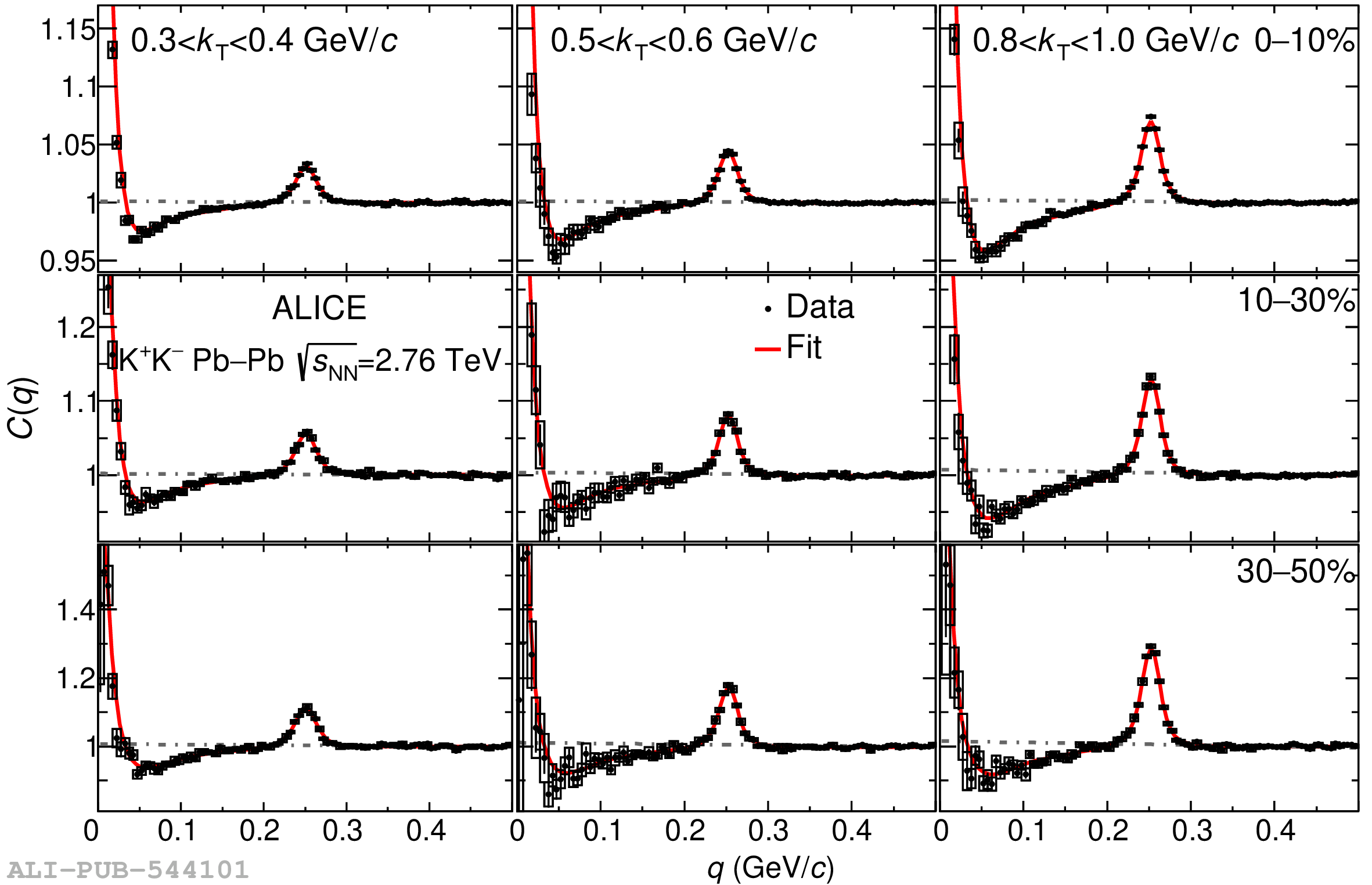# Figure 2

 The K$^+$K$^-$ experimental correlation functions corrected for non-flat baselines according to Eq. (14) as a function of pair relative momentum $q$. The CFs are presented in threecentrality classes (rows): 0-10%, 10-30%, and 30-50% and three pair transverse momentum $k_{\rm T}$ bins (columns): (0.3-0.4), (0.5-0.6) and (0.8-1.0) GeV/$c$. Statistical (bars) and systematic (boxes) uncertainties are shown. The red line shows thefit of the CF with the Lednický—Lyuboshitz parametrization (Eq. (13)) using free parameters (mass and couplings) for $f_0$(980) and Achasov  parameters  for $a_0$(980) in the 0 < $q$ < 0.5 GeV/c range. The dashed-dotted lines correspond to the baseline from Eq. (14).